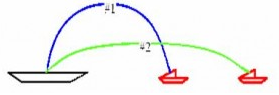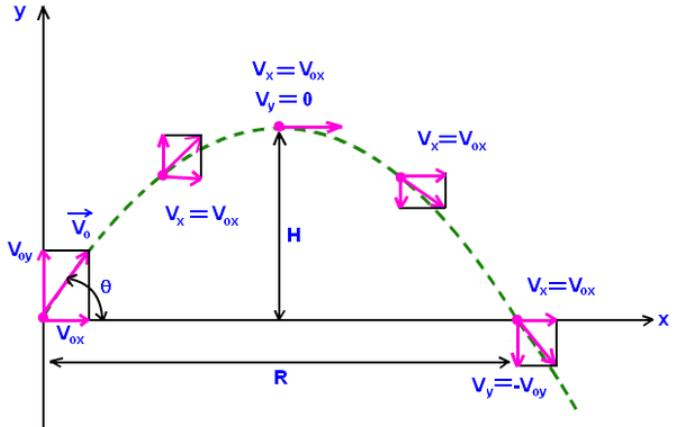Courses

# Test: Projectile Motion

## 10 Questions MCQ Test Physics For JEE | Test: Projectile Motion

Description
This mock test of Test: Projectile Motion for JEE helps you for every JEE entrance exam. This contains 10 Multiple Choice Questions for JEE Test: Projectile Motion (mcq) to study with solutions a complete question bank. The solved questions answers in this Test: Projectile Motion quiz give you a good mix of easy questions and tough questions. JEE students definitely take this Test: Projectile Motion exercise for a better result in the exam. You can find other Test: Projectile Motion extra questions, long questions & short questions for JEE on EduRev as well by searching above.
QUESTION: 1

### Which one of the following is not an example of projectile?

Solution:

Projectile motion is a natural phenomenon it is due to the gravity in earth surface .it doesn't need any external force .The flight seems to be same as an projectile motion but it differs as an external force is applied which is the aircraft engine which pulls the craft forward and hence oppose the force of gravity.

QUESTION: 2

### A battle ship simultaneously fires two shells at enemy ships. Both are fired with the same speed but with different directions as shown. If the shells follow the parabolic trajectories shown, which ship gets hit first?Solution:

Ship 2 gets hit first. The time of flight only depends on the y-component of motion, not the x-component.  The higher you throw something up in the air, the more time it spends in the air.  It can also be shown from equation-

y=v0y t – ½ g t2=0

v0y for projectile μ1 is greater than for μ2.

QUESTION: 3

### If an object is dropped through the window of a fast running train. Then

Solution:

When an object is still held it is the part of a system in which the train is moving, once it is left it still has the same velocity as the train for a person from ground. Thus as the velocity is horizontal and acceleration is vertical, it would follow a parabolic trajectory.

QUESTION: 4

Which statement is true for a ball thrown at 20 degrees with the horizontal, when it is at the highest point in its trajectory?

Solution:At the highest point in its trajectory, the acceleration (g) is acting in downward direction, whereas velocity of the projectile is only in the X (horizontal) direction.

QUESTION: 5

How many directions are possible for the same horizontal range?

Solution:

We know that the horizontal range for any projectile motion let say R = 2u2.sin 2a /g
Where u is initial speed, and a is the angle at which the particle is thrown, which is responsible for direction. So in between the possible range of a that is 0 - 90, there are maximum two equal values of sin 2a, thus the maximum number of directions for the same or equal range are 2.

QUESTION: 6

A body is thrown with a velocity of 10m/s at an angle of 60 degrees with the horizontal. Its velocity at the highest point is:

Solution:

At the highest point, its vertical velocity will become zero. Hence the only left velocity is horizontal which remains unchanged and is equal to 10 cos 60 = 5m/s

QUESTION: 7

The quantity which remains unchanged during the flight of an oblique projectile is

Solution:

As there is no horizontal acceleration is present in oblique projection horizontal component of velocity is constant

QUESTION: 8

At the point of maximum height, the acceleration is:

Solution:

At a point of maximum height, the derivative of displacement i.e. velocity is zero but as the gravitational acceleration is equal at all near points to the surface of earth, acceleration at maximum height is still equal to g.

QUESTION: 9

At what angle should a projectile with initial velocity ‘v’ be thrown, so that it achieves its maximum range?

Solution:

Sine of an angle has maximum value 1 when the angle is 90 degree Rmaxis obtained when 2ø = 90 degreeorø= 45 degree

QUESTION: 10

When a projectile is thrown up at an angle θ to the ground, the time taken by it to rise and to fall are related as

Solution:

The time of fall or rise for a projectile depends upon its vertical component of initial velocity and the vertical acceleration. As the acceleration does not change and the motion of path is identical for the rise phase and the fall phase, we can easily deduce that time taken is also the same.